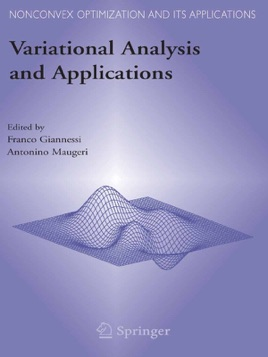• \$349.99

## Publisher Description

This book discusses a new discipline, variational analysis, which contains the calculus of variations, differential calculus, optimization, and variational inequalities. To such classic branches of mathematics, variational analysis provides a uniform theoretical base that represents a powerful tool for the applications. The contributors are among the best experts in the field.

Audience

The target audience of this book includes scholars in mathematics (especially those in mathematical analysis), mathematical physics and applied mathematics, calculus of variations, optimization and operations research, industrial mathematics, structural engineering, and statistics and economics.